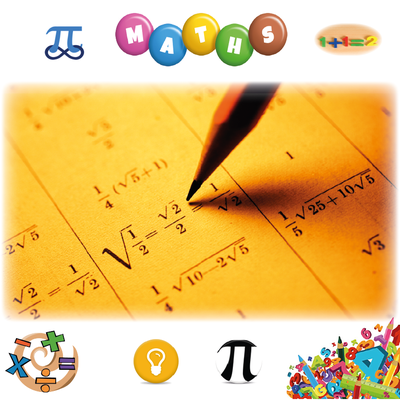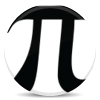## Learn ﻿﻿﻿Mathematics

An easy way to learn Mathematics online for free. Learn Maths Basics & Prealgebra; Geometry, Algebra & Trigonometry; Precalculus, Calculus & much more through this very simple course. Multiple Choice Tests. Thanks to Mem creators, Contributors & Users.
64.7k learners 286h avg duration### 100 x 100  Multiplication Table

30.1k 81h### Maths GCSE

16.5k 50mMaths

### Learn Times Tables

16.2k 1h### Multiplication Tables

13.7k 2hMaths

### Learn ﻿﻿﻿﻿﻿﻿﻿Statistics

12k 23h### 'Basic Trigonometric Identities'

11.9k 35m### Probability Theory and Statistics (Introduction)

6.77k 7h### A level Edexcel Maths and Further Maths

6.16k 2h### 100 Perfect Squares

6.14k 2h### 150 Digits of Pi

5.42k 37m### Edexcel D1 Definitions

4.84k 25m### General Maths (Methods) CAS VCE - Trigonometry

3.73k 21mMaths

### 1 - 144 Times Tables! Multiplication and division

3.59k 5hMaths

### Conversions

3.25k 19m3.1k 22m